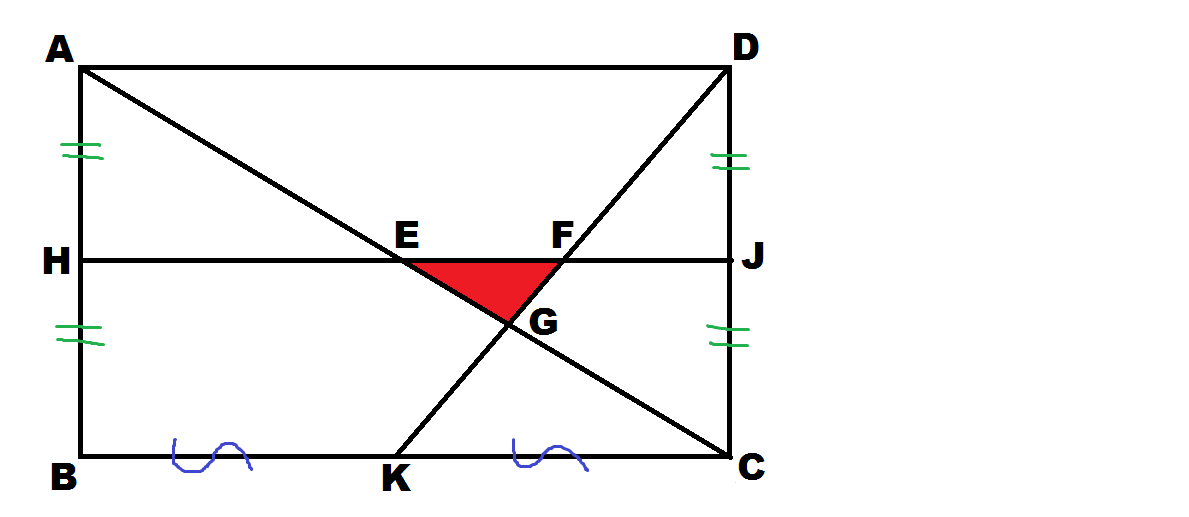# That red triangle is so isolated!In rectangle $ABCD$, $K,H,J$ are the mid-points of $BC,AB,DC$ respectively. Line-segments $AC,HJ,DK$ bound a red triangle $EFG$. Find the ratio of the area of the big rectangle to the area of red triangle. Give your answer to correct 2 decimal places.

×